###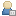Show Posts

This section allows you to view all posts made by this member. Note that you can only see posts made in areas you currently have access to.

### Messages - Dr_D

Pages:  2 3 4 5 6 7
1
##### Freebasic / Re: Need Help Waving PIC
« on: May 21, 2012 »
Hey guys... long time no see.

Cod1ngFreak, I was messing around with FreeBASIC a few years ago and made something to that effect. It's probably not what you expect... and not really what the functionality was designed for, in my opinion, but it does use a sine wave to manipulate an image as in your ascii drawing.  Hope it's useful.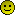2
##### General coding questions / Re: Aligning a matrix with a polygon normal(axis-angle)
« on: September 30, 2011 »
Thanks, rain_storm... I'll test it out when I have a chance. Thanks Jim, and you're right, but that isn't actually the problem. Somehow my matrix was becoming non-orthogonal.

Rel, it's that game I've been working on with Mysoft. I jhaven't actually worked on it for a while though. Been working on that Squealers mini-golf game.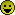3
##### General coding questions / Re: Aligning a matrix with a polygon normal(axis-angle)
« on: September 23, 2011 »
Hey guys... I haven't worked on it for a while, but I was wondering if anyone had any other suggestions or spots anything funky in my code?

4
##### General coding questions / Re: Aligning a matrix with a polygon normal(axis-angle)
« on: August 28, 2011 »
That method is what I tried using in the original test. Actually, here's my axis-angle matrix creation function:

Code: [Select]
`sub mat33_axisangle( matrix as number ptr, v as vec3 ptr, angle as number ) dim as number tc = cos(angle) dim as number ts = sin(angle) dim as number tt = 1.0 - tc vec3_normalize( v ) with *v matrix  = tc + .x*.x*tt matrix  = tc + .y*.y*tt matrix = tc + .z*.z*tt dim as number tmp1 = .x*.y*tt dim as number tmp2 = .z*ts matrix = tmp1 + tmp2 matrix = tmp1 - tmp2 tmp1 = .x*.z*tt tmp2 = .y*ts matrix = tmp1 - tmp2 matrix = tmp1 + tmp2 tmp1 = .y*.z*tt tmp2 = .x*ts matrix = tmp1 + tmp2 matrix = tmp1 - tmp2 end withend sub`

It seems to fail at certain angles though... it's hard to explain. I guess it's possible that I'm using a bad method to find the axis and angle? I had this method working a long time ago for a go-kart game, but I've lost that code and apparently the knowledge along with it. :p

Anyway... this is how I calculate the axis and angle:

Code: [Select]
`dim as vec3 r, u, axis u = poly.normal 'polygon normalr = vIntersection 'intersection pointvec3_normalize( @r )vec3_cross( @axis, @r, @u )  'return the cross product of the normal and the normalized intersection pointdim as single rot = vec3_dot( @u, @r ) 'calculate the angle between themmat44_axisangle( @tMatrix(0), @axis, rot )mat44_loadidentity( @oMatrix(0) )oMatrix(12)=position.xoMatrix(13)=position.yoMatrix(14)=position.zmat44_mul_mat44( @oMatrix(0), @tMatrix(0) )mat44_rotate( @oMatrix(0), 0, angle, 0 )`

5
##### General coding questions / Re: Aligning a matrix with a polygon normal(axis-angle)
« on: August 27, 2011 »
Well. I actually do need to be able to set it from any orientation. Do you know of the old driving game called Stunts? My friend and I are re-making that for Nintendo DS. So... it can be pretty much any arbitrary vector for up.

6
##### General coding questions / Re: Aligning a matrix with a polygon normal(axis-angle)
« on: August 27, 2011 »
Yep, you understand it right. However, I'm still not able to get it aligned properly with that technique. I've even tried rotating a point about the up vector at whatever angle my vehicle's current steer angle is and still no dice. That's probably where it's failing... How exactly would you go about constructing the new forward vector?

7
##### General chat / Re: Atari declares war on home-brew
« on: August 26, 2011 »
lol... very clever.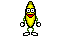8
##### General coding questions / Aligning a matrix with a polygon normal(axis-angle)
« on: August 25, 2011 »
Hey guys. I'm having a matrix problem here. I'm using FreeBASIC, but as this is a general math problem I put it here. Anyway... here we go. I need to align a 3d object with a polygon normal. To be more precise, I need to align the object's up vector with the polygon normal, while still allowing free rotation about it. In general this isn't a problem... if the current rotation about the up vector isn't important. For what I'm trying to do here here though, it is.

For instance, I can copy the polygon's normal to the up vector. I can then subtract the midpoint from one of the polygon's vertices and normalize to get the right vector . Then I can take the cross product of those two to get the forward vector and complete an orthogonal rotation matrix. All that sounds fine... but not when you're simulating a vehicle. I need to be able to rotate freely about up and keep the angle consistent when a new collision is detected. I tried with an axis-angle approach, but something seems to be off. Has anyone ever dealt with this before? I've been testing different methods for about a week now and it's starting to drive me nuts. :p

9
##### General chat / Re: Atari declares war on home-brew
« on: August 24, 2011 »
Man, this is just sad. They should be elated that people are still messing around with that old system!

10
##### Freebasic / Re: House on the Hill
« on: April 21, 2011 »
I love the intro... that sets up a great story for a game. I'm not into text adventures much, but this kind of story might suck me in because I love this genre. Keep it up man!11
##### Freebasic / Re: Stereoscopic geometry test
« on: October 31, 2010 »
Shit, that's awesome man!

12
##### Freebasic / Re: Clip coordinates problem... or to put it better, the lack thereof.
« on: October 16, 2010 »
Thanks for the lengthy, detailed response dude. You rock! I had a similar idea, but didn't know if it was even worth going to all the trouble to test. I will have to modify my current system a bit to make it work with edges, as it now uses and indexed vertex system.

13
##### Freebasic / Re: Clip coordinates problem... or to put it better, the lack thereof.
« on: October 14, 2010 »
Quote from: Jim
(the scanline renderer can skip the off-to-the-left-pixels with a quick multiply and early-out on the off-to-the-right ones)

Actually, that's what I'm doing now... it does a multiply for pixels off screen to the top as well... it just muls everything by the negative value of y, where y is off screen. There aren't many check in the triangle rsaterizers now, but there are enough to keep someone with my limited asm skills from getting both loops in. That sounds very tempting to totally remove those checks and muls from the triangles. I'm still working, but I have to admit I'm having trouble even getting things clamped to the near plane... or 0, for that matter right now.

Ok... so here's a question with substance. If I clip vertices to the near plane, how do I determine which vertices should actually be drawn from there as anything behind the eye will have a z value of zNear? You mentioned a bit operation. My guess is you're testing if all 3 vertices are behind the eye and then skip that triangle completely if true? I'm using an indexed array setup for this, wavefront(OBJ), so that might not be the best storage method for this method of rendering.

14
##### Freebasic / Re: Clip coordinates problem... or to put it better, the lack thereof.
« on: October 13, 2010 »
Thanks for the links & stuff guys. For the most part, I think I should be able to get away with just clipping to the near plane. I'm not really worried about full frustum clipping... unless I run into more problems later. Theoretically, it should be ok as the triangle functions wont ever allow and out of bounds writing. Anyway, reading about that algorithm helps a lot too. Thanks again guys... if I make anything cool enough I'll post it.15
##### Freebasic / Clip coordinates problem... or to put it better, the lack thereof.
« on: October 12, 2010 »
Ok, so I've been following the math on this page, and trying to recreate it all on the CPU, even as will be exponentially slower. I'm not concerened about that, I'm just trying to become a better all around graphics programmer. Anyway, I don't understand exactly how they're handling the clip coordinates. What I'm doing... is simply missing something, as I have vertices that are being projected to infinity, where there is no chance to clip those when they are close to the eye, as they are wildly out of range. Here is some code... if that may help anyone help me. Thanks guys.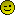Code: [Select]
`   dim as single w = any dim as vec4f pvec  'clip coordinates dim as vec3f ndvec 'normalized device coordinates for i as integer = 0 to Model->max_vertices - 1 with model->tvertices[i] lx = .x ly = .y lz = .z end with 'generating clip coordinates here... doesn't seem quite clear to me...                'mar() = view matrix * model matrix with pvec .x = lx*mar(0) + ly*mar(4) + lz*mar(8) + mar(12) .y = lx*mar(1) + ly*mar(5) + lz*mar(9) + mar(13) .z = lx*mar(2) + ly*mar(6) + lz*mar(10) + mar(14) .w = lx*mar(3) + ly*mar(7) + lz*mar(11) + mar(15) end with                                'just keeping the reciprocal under control... if pvec.w > 0 then w = 1f / pvec.w else w = 1f end if 'normalized device coordinates ndvec.x = pvec.x * w ndvec.y = pvec.y * w ndvec.z = pvec.z * w 'screen coordinates calculated now and stored back in model's pvertices array                'everything is still raw in here for clarity and my own sanity...                'tscrh = screen width, tscrh = screen height with model->pvertices[i] .x = (tscrw * ndvec.x) + (ndvec.x + tscrw) .y = top-((tscrh * ndvec.y) + (ndvec.y + tscrh)) .z = ((zFar-zNear)/2) * ndvec.z + ((zFar+zNear)/2) end with next`

16
##### General chat / Re: 0xFFFFFFFFFFFFFFFFFFFF Karma for hellfire!
« on: September 21, 2010 »
Cool! Now make more awesome stuff! :p

17
##### Freebasic / Re: Stereoscopic geometry test
« on: July 24, 2010 »
Heya... I messed around with this a bit a while back. It's pretty fun. I think I need to change the colors in yours for my glasses to work because I have those old style red/blue ones. Anyway, here's the thing I made if you wanna have a look. :p

I tried a few things, but where I got the best results was this: Render each eye to a separate image, fudge them over a bit and then blend. So, I moved the left render right by n pixels, and moved the right render left by n pixels.That fudging thing wasn't based on anything except messing around with images in Photoshop. It does seem to look a bit better to me, but that may just be me.

18
##### Freebasic / Re: Perspective correct texture mapping problems...
« on: March 05, 2010 »
Damn, I never noticed that.. I guess that's what Stonemonkey mentioned. It makes sense now. :p

19
##### Freebasic / Re: Perspective correct texture mapping problems...
« on: March 04, 2010 »
Yeah, that rounding would be different since fb's integer rounding by default only scales up when it's >= .5. I've modified that bit to use the ceil function, making it consistent across all variables. I've also used ceil for converting the y loops to integer, but it's still acting weird. I have to find where that dn calculation is drifting to infinity... I'm sure that's it, it so close now.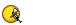20
##### Freebasic / Re: Perspective correct texture mapping problems...
« on: March 04, 2010 »
Man, this is crazy... Mine still doesn't work properly. I understand what's going on, or so I think, but it makes no sense for it to do this... it almost works, damn it. Can you see what's going on? I even switched to use reciprocals for the slope calculations, as yours does. It wont matter right now, but it's a nice optimization for the one with actual texturing and shading. Thanks again for the help.Code: [Select]
`function sub_pix( byval inval as single ) as single return 1f - (ceil(inval) - inval)end functionsub triangle( byref dst as FB.IMAGE ptr = 0, byref p1 as vec2f, byref p2 as vec2f, byref p3 as vec2f, byval col as uinteger ) dim as uinteger ptr dstptr, dptr1 dim as integer sx, ex dim as integer dw,dh, dpitch, dbpp dim as single d1, d2, d3, lx, rx, invheight if dst = 0 then dstptr = screenptr screeninfo dw,dh,,dbpp, dpitch else dstptr = cast( uinteger ptr, dst + 1) dw = dst->width dh = dst->height dbpp = dst->bpp dpitch = dst->pitch end if 'reverse for normal coordinate system dim as single y1 = (dh\pix_size) - p1.y dim as single y2 = (dh\pix_size) - p2.y dim as single y3 = (dh\pix_size) - p3.y dim as single x1 = p1.x dim as single x2 = p2.x dim as single x3 = p3.x if y2 < y1 then swap y1, y2 swap x1, x2 end if if y3 < y1 then swap y3, y1 swap x3, x1 end if if y3 < y2 then swap y3, y2 swap x3, x2 end if if y2>y1 then invheight = 1f/(y2-y1) else invheight = 1f end if d1 = (x2 - x1) * invheight if y3<>y2 then invheight = 1f/(y3-y2) else invheight = 1f end if d2 = (x3 - x2) * invheight if y1<>y3 then invheight = 1f/(y1-y3) else invheight = 1f end if d3 = (x1 - x3) * invheight lx = x1 + (d1*sub_pix(y1)) rx = x1 + (d3*sub_pix(y3)) locate 1,1 print d1, d1, d3 for y as integer = y1 to y2-1 if y>-1 and y<dh then sx = ceil(lx) ex = ceil(rx) if sx>ex then swap sx,ex end if if sx<0 then sx = 0 end if if ex>dw-1 then ex=dw-1 end if dptr1 = cast(uinteger ptr, cast(ubyte ptr, dstptr) + y * dpitch + sx * dbpp) for x as integer = sx to ex '*dptr1 = col dptr1+=1 line(x*pix_size,y*pix_size)-step(pix_size-1,pix_size-1),col,bf next end if lx += d1 rx += d3 'y+=1 next lx = x2 + (d2*sub_pix(y2)) 'rx = constant slope for y as integer = y2 to y3 if y>-1 and y<dh then sx = ceil(lx) ex = ceil(rx) if sx>ex then swap sx,ex end if if sx<0 then sx = 0 end if if ex>dw-1 then ex=dw-1 end if 'x = sx dptr1 = cast(uinteger ptr, cast(ubyte ptr, dstptr) + y * dpitch + sx * dbpp) for x as integer = sx to ex '*dptr1 = col dptr1+=1 line(x*pix_size,y*pix_size)-step(pix_size-1,pix_size-1),col,bf next end if lx += d2 rx += d3 nextend sub`

Pages:  2 3 4 5 6 7